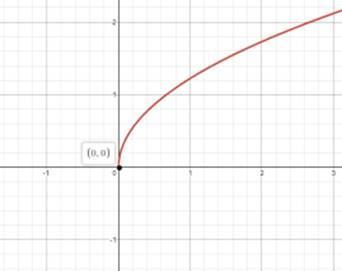# the graph of given equation when x lies from 0 to 100.### Precalculus: Mathematics for Calcu...

6th Edition
Stewart + 5 others
Publisher: Cengage Learning
ISBN: 9780840068071### Precalculus: Mathematics for Calcu...

6th Edition
Stewart + 5 others
Publisher: Cengage Learning
ISBN: 9780840068071

#### Solutions

Chapter 1.9, Problem 73E

(a)

To determine

## To sketch:the graph of given equation when x lies from 0 to 100.

Expert Solution

The graph shows that the more is the height of the person more far the person can see the farthest distance.

### Explanation of Solution

Given:

y=1.5x+(x5280)2 .

Concept used:

Desmos graphing calculator is used here to plot the graph.

Calculation:

Work as shown below, follow the steps:

(a) graph the equation y=1.5x+(x5280)2 on a graphing calculator as shown in the following picture:The graph shows that the more is the height of the person more far the person can see the farthest distance.

(b)

To determine

### To find:the height of the person above the sea level when person can bale to see 10 mi.

Expert Solution

The two graphs intersect at the point (66.667,10) so for someone to be able to see 10mi far he must be x=66.667ft above the sea level.

### Explanation of Solution

Given: y=1.5x+(x5280)2 .

Concept used:

Desmos graphing calculator is used here to plot the graph.

Calculation:

2. (b) in order to answer this question it must find the value of x such that

y=10mi .

In the graph of this equation above graph the horizontal line y=10 as shown in the following picture:The two graphs intersect at the point (66.667,10) so for someone to be able to see 10mi far he must be x=66.667ft above the see level.

### Have a homework question?

Subscribe to bartleby learn! Ask subject matter experts 30 homework questions each month. Plus, you’ll have access to millions of step-by-step textbook answers!